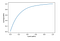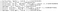# A Complete Guide to Exploratory Data Analysis on Structured Data

Exploratory Data Analysis (EDA) is used to analyze and understand data sets to unearth hidden patterns and insights. In Data Science, this is often employed as the preliminary step in any Advanced Analytics task.

There are numerous objectives of conducting an EDA:

● Maximizing the insight that can be gained from a data set.

● Uncovering the underlying structure.

● Extracting important variables.

● Detecting outliers and anomalies.

● Testing underlying assumptions.

● Developing and testing hypothesis.

● Determining the optimal factor settings.

If done properly, EDA answers following core questions:

1. How do we dissect a data set?
2. What do we look for in Data?
3. How do we look at Data ?
4. How do we interpret Data ?

The objective of this article is not to explain these techniques in detail, but to provide a guideline into which technique to apply when. The types of techniques that one might wish to apply would depend on the type of data and the objective of the task. We can divide the data into two types: structured and unstructured. In this article, we will only be focusing on structured data.

EDA Matrix

The following matrix can be used as a framework when selecting the EDA techniques based on the type of EDA and whether the requirement is a graphical or a non-graphical representation.

Each of the techniques listed in this matrix will be discussed with examples in the following section.

Types of EDA

In this section, a marketing campaign dataset available in the Kaggle website was used to discuss relevant techniques with examples of their usage. This data set contains customer information, such as demographic information and purchase history, and their responses to a particular marketing campaign. We will conduct an analysis using this dataset to understand the dataset and derive useful insights using various EDA techniques.

1. Univariate Analysis

Univariate Analysis is the simplest form of analyzing data. It does not deal with causes or relationships, and its main purpose is to describe data by summarizing and finding patterns, and drawing conclusions from the findings. Few techniques under this analysis are:

● Frequency

● Central Tendency Measures (mean, median, and mode)

● Dispersion/Spread of Data (range, minimum, maximum, quartiles, variance, and standard deviation)

Moreover, frequency distribution tables, histograms, pie charts, and bar charts can be used to visualize these patterns.

2. Multivariate Analysis

The main objectives of Multivariate Analysis are,

● Identifying correlation and dependencies of variables

● Constructing and testing a Hypothesis

● Gaining insights for feature selection and engineering

Correlation matrices, line charts, scatterplots and heatmaps can be used to visualize these patterns.

3. Clustering

Clustering reduces a large data set to meaningful subgroups. The division is based on the similarity of the objects across a set of specified characteristics.

Four main rules for developing clusters,

● Should be different

● Should be reachable

● Should be measurable

● Should be profitable (large enough to be noteworthy)

4. Pareto Analysis

Commonly known as the ‘80/20 rule’, Pareto Analysis statistically separates a limited number of input factors based on which has the greatest impact on the outcome. This principle is applicable for many day-to-day scenarios in the real world.

Vilfred Pareto, an Italian economist and sociologist introduced this theory in 1896 by demonstrating that 80% of the wealth of Italy was distributed among 20% of the population, and the remaining 20% of the wealth was distributed among the other 80% of the population.80% of the revenue is caused by ~30% of the customers

5. Frequent Itemset Mining

Characterized by ‘thinking outside the basket’, this technique is widely used in Market Basket Analysis. This is a rule-based Machine Learning method for discovering relations between variables in large databases by analyzing patterns or co-occurrences. Apriori and FP-Growth are two common algorithms used for Association Rule Mining. When applying Frequent Itemset Mining in EDA, similar to how items bought together in a particular transaction becomes the list of items in that market basket, the set of attributes in a record becomes the list of items.Frequent Attributes of high value customers using FP-Growth (Min Support = 0.2 and Min Confidence = 0.7)

6. Outlier Detection

This is the process of identifying unexpected items or events in data sets, which differ from the norm. However, anomalous data can indicate critical incidents, such as a technical glitch, or potential opportunities, for instance a change in consumer behavior, or simply erroneous data. Even though there are many methods to identify outliers, it is important to apply domain knowledge to describe these outliers.

Graphical Methods:

● Boxplots

● Scatter plots

Mathematical Functions:

● IQR

● Z-score

Machine Learning Models:

● One class SVM

● DBSCAN (Clustering Method)

Volatility

Volatility is a commonly used technique in Stock Analysis. This is an ideal analysis of variation for time series data. This is a calculation that says how much data changes/varies during each interval. There are many techniques of calculating Volatility. The following example shows the historic volatility calculation for a sample stock data set at a daily value.

Conclusion

There are numerous EDA techniques. However, it might be impractical to conduct all these Analyses during the short life cycle of a Data Science Project. Moreover, these techniques might not be useful for all analytics tasks. Hence, it is important to select which technique is the most suitable based on the problems and the dataset at hand.

Written by: Chamodi Adikaram, Data Scientist.

OCTAVE, the John Keells Group Centre of Excellence for Data and Advanced Analytics, is the cornerstone of the Group’s data-driven decision making.

## More from OCTAVE - John Keells Group

OCTAVE, the John Keells Group Centre of Excellence for Data and Advanced Analytics, is the cornerstone of the Group’s data-driven decision making.